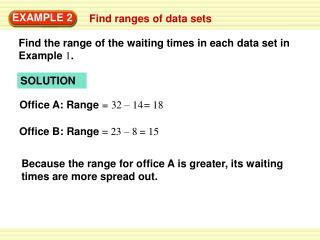DownloadDownload PresentationEXAMPLE 2

# EXAMPLE 2

Download Presentation## EXAMPLE 2

- - - - - - - - - - - - - - - - - - - - - - - - - - - E N D - - - - - - - - - - - - - - - - - - - - - - - - - - -
##### Presentation Transcript

1. EXAMPLE 2 Find ranges of data sets Find the range of the waiting times in each data set in Example 1. SOLUTION Office A: Range= 32 – 14 = 18 Office B: Range= 23 – 8 = 15 Because the range for office A is greater, its waiting times are more spread out.

2. The correct answer is D. ANSWER – (14 22)2 – + (17 22)2 290 Office A: = 5.7 = 9 9 ... – + + (32 22)2 ... – – (8 16)2+ (11 16)2+ + (23 16)2 – Office B: 182 = 4.5 9 = 9 EXAMPLE 3 Standardized Test Practice SOLUTION

3. Standard deviation ... (x1 – x)2 + (x2 – x)2 + + (xn – x)2 ... = (2 – 7.4)2 + (4– 7.4)2 + + (15 – 7.4)2 = n 10 148.4 = 10 for Examples 2 and 3 GUIDED PRACTICE 2.Find the range and standard deviation of the data set in Guided Practice Exercise 1 on page 745. SOLUTION Range= 15 – 2 = 13 = 3.8